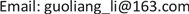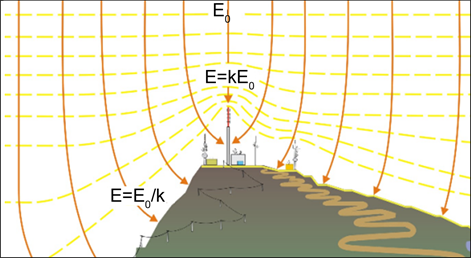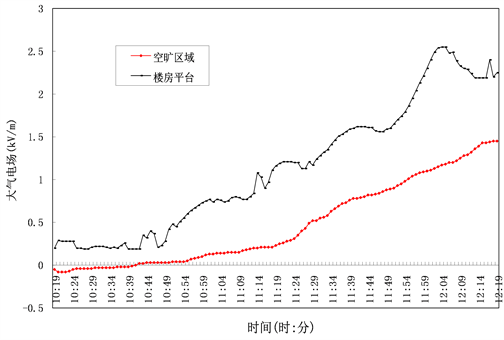﻿ 大气电场数据误差的影响分析 Analysis of the Atmospheric Electric Field Data Error Impact

Vol.07 No.06(2017), Article ID:22982,6 pages
10.12677/AG.2017.76078

Analysis of the Atmospheric Electric Field Data Error Impact

Guoliang Li1, Jun Li2, Xiang Li1, Ying Pi1, Xin Li1

1Hubei Lightning Protection Center, Wuhan Hubei

2Hubei Meteorological Information and Technical Support Center, Wuhan HubeiReceived: Nov. 20th, 2017; accepted: Dec. 4th, 2017; published: Dec. 11th, 2017ABSTRACT

For the study of atmospheric electric field data error, solving the problem of atmospheric electric field instrument data consistency, this paper simulated different size of the cube instead of the real buildings, high voltage wire, metal rods, trees and so on with Ansoft Maxwell electromagnetic field simulation software. Analysis shows that the terrain environment and instrument installation height on the ground have a great influence on the ground atmospheric electric field measurement results, which makes the atmospheric electric field instrument data lack of comparability between each observation point. It is concluded that the installation of the electric field instrument must consider environmental factors, it away from the obstacle distance shall not be less than 3 times of the barrier height and 2 times of the width, considering the influence of the barrier height c and width depth ratio a/b at the same time. The influence of the height and environmental equipment can use linear fitting to correct with reference to the standard measurement data.

Keywords:Atmospheric Electric Field, Terrain Environment

1湖北省防雷中心，湖北 武汉

2湖北省气象信息与技术保障中心，湖北 武汉1. 引言

2 电场仪的测量原理

$E=\underset{i=1}{\overset{n}{\sum }}\frac{{Q}_{i}\cdot {H}_{i}}{2\text{π}{\epsilon }_{0}\cdot {\left({H}_{i}^{2}+{L}_{i}^{2}\right)}^{3/2}}$ (1)

3. 大气电场分布特征

3.1. 空间分布

3.2. 时间分布

4. 设备高度误差分析

$\varphi =-{E}_{0}y+\frac{1}{4\text{π}{\epsilon }_{0}}\underset{0}{\overset{h}{\int }}\frac{\rho \text{d}y}{\sqrt{{x}^{2}+{y}^{2}}}+\frac{Q}{4\text{π}{\epsilon }_{0}}\left[\frac{1}{\sqrt{{x}^{2}+{\left(y-h\right)}^{2}}}\right]$ (2)

$E\left(x,y\right)=-\nabla \Phi \left(x,y\right)=-\frac{1}{4\text{π}{\epsilon }_{0}}\left\{\frac{\rho }{x\sqrt{{h}^{2}+{x}^{2}}}+\frac{Qx}{{\left[{x}^{2}+{\left(y-h\right)}^{2}\right]}^{3/2}}\right\}i+\left\{{E}_{0}-\frac{Q\left(y-h\right)}{2\text{π}{\epsilon }_{0}{\left[{x}^{2}+{\left(y-h\right)}^{2}\right]}^{3/2}}\right\}j$ (3)

5 设备环境误差分析

k为电场仪安装的地形参数，当地形参数较小时(一般1 < k < 3)，可通过配置设备的地形参数进行纠正而不影响探测精度；当k > 3时，通过调整电场仪的地形参数来校准就会损失探测精度，因此要求电场仪尽量安装在空旷、平坦、周边没有高大物体遮挡的地面，一般要求探头所在位置对天仰角需要大于120o。为方便研究，将地面上凸出的建筑物、高压线、金属杆、树木等简化为立方体，通过对立方体周围的大气电场进行了仿真分析，得出不同立方体的大小、距离、高度对大气电场影响。设研究空间只有一个外加的垂直向下的均匀电场E0，地面及凸出物的表面电位均为0，立方体的宽为a、高为c、深为b。目前场磨式大气电场仪只对垂直方向的电场进行监测，故只考虑垂直方向上大气电场测量值，设环境修正系数k = E/E0。其中：E为大气电场测量值，E0为实际大气电场值。用电磁场仿真软件Ansoft Maxwell进行仿真计算和分析发现：1)随着与立方体距离加大，立方体对近地表面电场产生的影响减小得很快，当离立方体的距离增加至立方体宽度和高度(a + c)时，电场受到该立方体的影响才会变得很小；2)若立方体的高度c大于宽度a，即立方体高度作为主要影响因子，地面电场的影响范围主要取决于高度c，即在与立方体距离大于3倍立方体高度区域，地表面电场几乎不再受立方体的影响。3)若立方体的高度c小于宽度a，此时地面电场的影响范围主要取决于a。即在与立方体距离大于2倍宽度a的区域，地表面大气电场几乎不再受立方体的影响；4)对于a ≤ c ≤ 3a的立方体模型，在计算其影响范围和不同位置处的修正系数时则需同时考虑高度c和宽深比a/b的影响，不同尺寸关系的模型其结果可能相差较大   。

6 电场测量数据订正

6.1 对比分析Figure 1. Distribution of atmospheric electric field around buildings

6.2. 效果检验Figure 2. Monitoring values of atmospheric electric field in different environments

7. 结论

1) 测量误差的主要来源有设备误差和环境误差。环境误差主要与设备安装高度和安装环境有关；

2) 电荷量多少和传感器高度均会影响电场强度，在雷暴天气下的误差值比晴天要大；

3) 大气电场仪离建筑物、高压线、金属杆、树木等越远、影响和误差值越小；

4) 若障碍物的高度大于宽度时，电场仪距离障碍物的距离应大于障碍物高度的3倍；若障碍物的高度小于宽度时，电场仪距离障碍物的距离应大于障碍物宽度的2倍；

5) 电场仪安装位置要充分考虑距障碍物的距离，同时考虑障碍物高度c和宽深比a/b的影响。

6) 将所有电场仪数据与环境好的电场仪进行统计分析，通过最小二乘法找出相互关系，对所有电场仪测量数据进行修正，实现数据的一致性。

Analysis of the Atmospheric Electric Field Data Error Impact[J]. 地球科学前沿, 2017, 07(06): 772-777. http://dx.doi.org/10.12677/AG.2017.76078

1. 1. 李振亚, 肖稳安, 张其林, 等. 小波分析在雷击大气电场波形去噪分析中的应用[J]. 气象科学, 2014, 34(3): 282- 287.

2. 2. 郭秀峰, 谭涌波, 郭凤霞, 等. 建筑物尖端对大气电场畸变影响的数值计算[J]. 应用气象学报, 2013, 24(2): 189- 196.

3. 3. 行鸿彦, 张强, 徐伟, 等. 大气电场仪观测数据的海拔校正及联网[J]. 解放军理工大学学报: 自然科学版, 2014, 15(6): 591-597.

4. 4. 周璧华, 姜慧, 杨波, 等. 地物环境对地面大气电场测量的影响[J]. 电波科学学报, 2010(5): 839-844.

5. 5. 耿雪莹, 张其林, 刘明远. 地面建筑物 (群) 对雷暴云大气电场影响的模拟研究[J]. 气象科技, 2012, 40(5): 827- 833.

6. 6. 许强. 不同相对介电常数建筑物对大气电场畸变的研究[J]. 电瓷避雷器, 2017(3): 178-181.

7. 7. 周苑, 黄钰. 大气电场仪本身对电场的影响和修正[J]. 自然科学:文摘版, 2016(5): 00239-00239.

8. 8. 柴健, 张谦. 雷暴天气下建筑物对大气电场测量的影响[J]. 解放军理工大学自然科学版, 2017, 18(1): 56-60.

9. 9. 杨超, 曾金全, 洪志敏, 等. 厦门沿海近地层大气电场垂直变化规律[J]. 热带海洋学报, 2016, 35(1): 96-101.

10. 10. 陈渭民. 雷电学原理[M]. 第2版. 北京: 气象出版社, 2006.

11. 11. 张谦, 柴健, 黄克俭. 雷暴天气下建筑物周围电场畸变区域的研究分析[J]. 中国农学通报, 2016, 32(6): 145-150.

12. 12. 余蓉, 杜牧云, 杜九三. 大气电场仪资料的小波去噪研究[J]. 成都信息工程学院学报, 2016, 31(2): 175-179.

13. 13. 邓海利, 李莉, 赵京峰, 等. 单站式大气电场仪探测距离分析[J]. 安徽农业科学, 2015, 43(21): 222-224.

14. 14. 秦微, 张其林, 姜苏, 等. 基于大气电场资料的雷电临近预警研究[J]. 南京信息工程大学学报, 2016, 8(3): 247- 251.

15. 15. 刘畅, 靳小兵, 陆茂, 等. 成都地区晴天地面大气电场特征研究[C]//防雷减灾论坛. 2015.